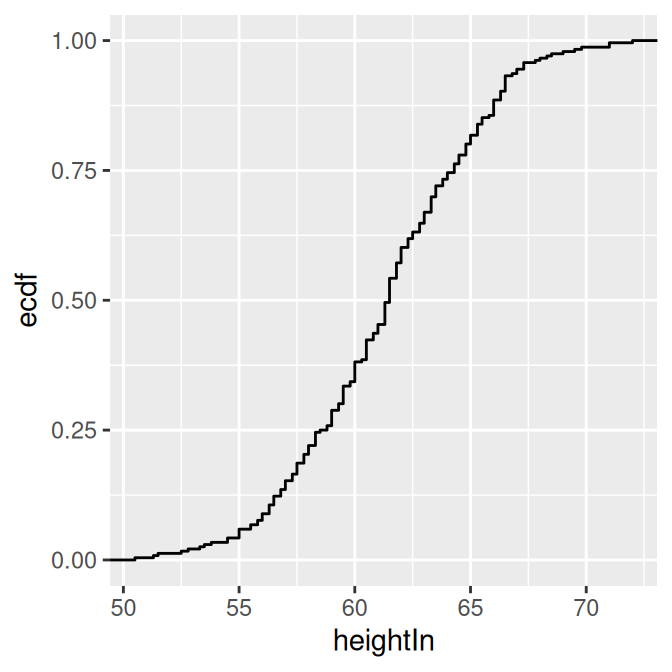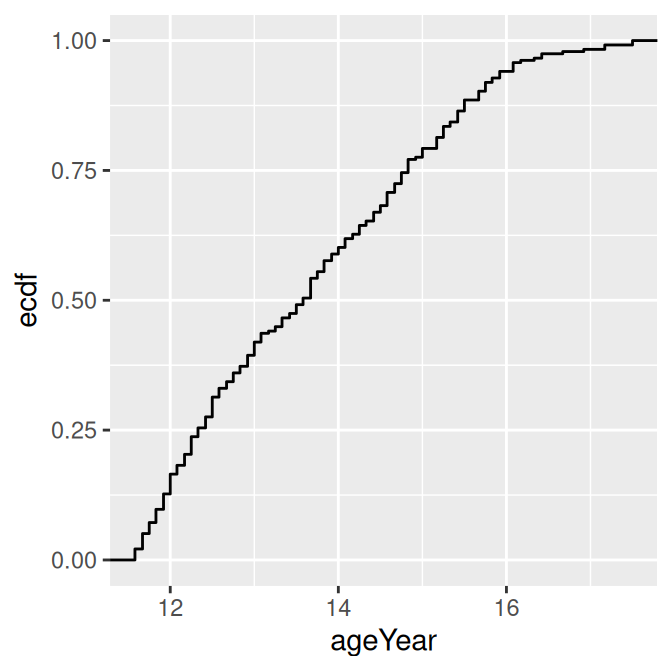## 13.14 Creating a Graph of an Empirical Cumulative Distribution Function

### 13.14.1 Problem

You want to graph the empirical cumulative distribution function (ECDF) of a data set.

### 13.14.2 Solution

Use `stat_ecdf()` (Figure 13.26):

``````library(gcookbook) # For the data set

# ecdf of heightIn
ggplot(heightweight, aes(x = heightIn)) +
stat_ecdf()

# ecdf of ageYear
ggplot(heightweight, aes(x = ageYear)) +
stat_ecdf()``````Figure 13.26: ECDF of height (left); ECDF of age (right)

### 13.14.3 Discussion

The ECDF shows what proportion of observations are at or below the given x value. Because it is empirical, the line takes a step up at each x value where there are one or more observations.